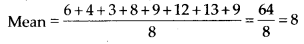# MP Board Class 7th Maths Solutions Chapter 3 Data Handling Ex 3.2

## MP Board Class 7th Maths Solutions Chapter 3 Data Handling Ex 3.2

Polynomial in ascending order calculator.

Question 1.
The scores in Mathematics test (out of 25) of 15 students are as follows:
19, 25, 23, 20, 9, 20, 15, 10, 5, 16, 25, 20, 24, 12, 20
Find the mode and median of this data. Are they same?
Solution:
Arranging the given scores in ascending order, we have 5, 9, 10, 12, 15, 16, 19, 20, 20, 20, 20, 23, 24, 25, 25
Mode of given data is that value of observation which occurs for the most number of times i.e., 20. 5.
Median of the given data is the middle observation when the data is arranged in ascending order i.e., 8th term = 20
Hence, mode of data = median of data.Question 2.
The runs scored in a cricket match by 11 players are as follows:
6, 15, 120, 50, 100, 80, 10, 15, 8, 10, 15
Find the mean, mode and median of this data. Are the three same?
Solution:
Arranging the given scores in an ascending order, we haveAs 15 occurs for the most number of times.
∴ Mode = 15
Median = Middle term = 15
∴ All three mean, mode and median are not same.Question 3.
The weights (in kg.) of 15 students of a class are: 38, 42, 35, 37, 45, 50, 32, 43, 43, 40, 36, 38, 43, 38, 47
(i) Find the mode and median of this data.
(ii) Is there more than one mode?
Solution:
Arranging the given weights in ascending order, we have 32, 35, 36, 37, 38, 38, 38, 40, 42, 43, 43, 43, 45,47, 50
(i) Mode = 38 and 43
Median = Middle term = 40
(ii) Yes, there are 2 modes for the given data i.e., 38 and 43.

Question 4.
Find the mode and median of the data: 13, 16, 12, 14, 19, 12, 14, 13, 14
Solution:
Arranging the given data in an ascending order, we have
12, 12, 13, 13, 14, 14, 14, 16, 19
14 occurs for the most number of times
∴ Mode = 14
Median = Middle observation = 14Question 5.
Tell whether the statement is true or false:
(i) The mode is always one of the numbers in a data.
(ii) The mean is one of the numbers in a data.
(iii) The median is always one of the numbers in a data.
(iv) The data 6,4,3,8,9,12,13,9 has mean 9.
Solution:
(i) True, as mode of a given data is that value of observation which occurs for the most number of times. Therefore, it is one of the observations given in the data.
(ii) False, because mean may or may not be one of the numbers in the data.
(iii) False, because median may or may not be one of the numbers in the data
(iv) False, because